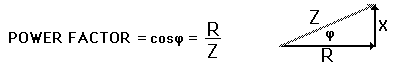Home » 2010 » November

# Monthly Archives: November 2010

## A question from Magnetism

“It is said that an iron piece behaves as a magnet when an external magnetic field is applied. Then otherwise why doesn’t it behave as a magnet even though the earth’s magnetic field is acting on it.”

Ans:

Every molecule of iron possess magnetic behavior. But in the absence of an external strong magnetic field, the individual molecular magnets form closed loops or they are arranged in a random fashion in such a way that the net magnetic effect is zero.

Since the magnetic dipole moments of all the molecular magnets inside the iron add up to zero, the iron rod does not respond to earth’s magnetic field like a magnet.

Further, the strength of earth’s magnetic field is not so large to magnetize a piece of iron. The coercivity of iron is also very less (it can easily lose any acquired magnetism)

However, steel buried in North South direction is found to acquire some magnetism.

## Power factor in AC circuits

“what is meant by power factor?”

Ans:

In AC circuits, Power factor is defined as the ratio of true power to apparent power.$Power Factor =\frac{True Power}{Apparent Power}$

If φ is the phase difference between E and I, then

True Power = EvIv cos φ and the Apparent Power is EvIv

Therefore,## Exhibition Models

Arjun send a request:

hai frnds pls suggest me a physics exhibition working model (conservation of energy)?

## What are the characteristics of a good balance?

Angana Asked: “What are the characteristics of a good balance?”

Ans: “There are two important characteristics of a good (true) balance.

1. The two arms must be of equal length
2. The two pans must be of equal weight.

## A question from Kinematics : 100m long a train passing a 40m long bridge in 20.Find out the velocity of the train?

Mitu asked – “100m long a train passing a 40m long bridge in 20.Find out the velocity of the train?”

Ans: The total distance to be traveled by the train to completely traverse the bridge is 100 + 40 = 140 m

Time = 20 s

From the relation,$speed = \frac{distance}{time}$$speed = \frac{140}{20}=7m/s$

## Equations of Motion – Images for easy reuse

Here you can find the equations of motion in the form of images which you can use in your documents.$v = u + at$$S = ut + \frac{1}{2} at^{2}$$v^{2} = u^{2} + 2aS$

## Big Bang and the conservation of Mass

“During the big bang it is supposed that the small particles expanded in a very very small interval of time and there was nothing before that. If it is so, then how can it in be good agreement with law of conservation of mass?”

Ans:

After the Big Bang, the universe expanded rapidly. The big bang theory is not a theory which is completely resolved. There are many questions to be answered.

Further, mass alone is not conserved, it is mass+energy  which is conserved.

Energy can be converted into matter and vice versa. ($E=mc^{2}$)

Dark Energy and Dark Matter are two widely discussed concepts in association with the Big Bang. These correspond to the mass (matter) and energy which we cannot account for by calculations and theory.

Further, BIGBANG is not the only possible cause of the Birth of Universe. There are many other possibilities.

Big Bang theory originated after the discovery that the universe is expanding. SO if we think in reverse direction, we can imagine that the entire universe was confined to an infinitesimally small (Compared to the present size of the universe) space. This idea started the theoritization of the Big Bang.

(Comments and better answers are expected from visitors and experts visiting the site)

### Hits so far @ AskPhysics

• 2,274,296 hits